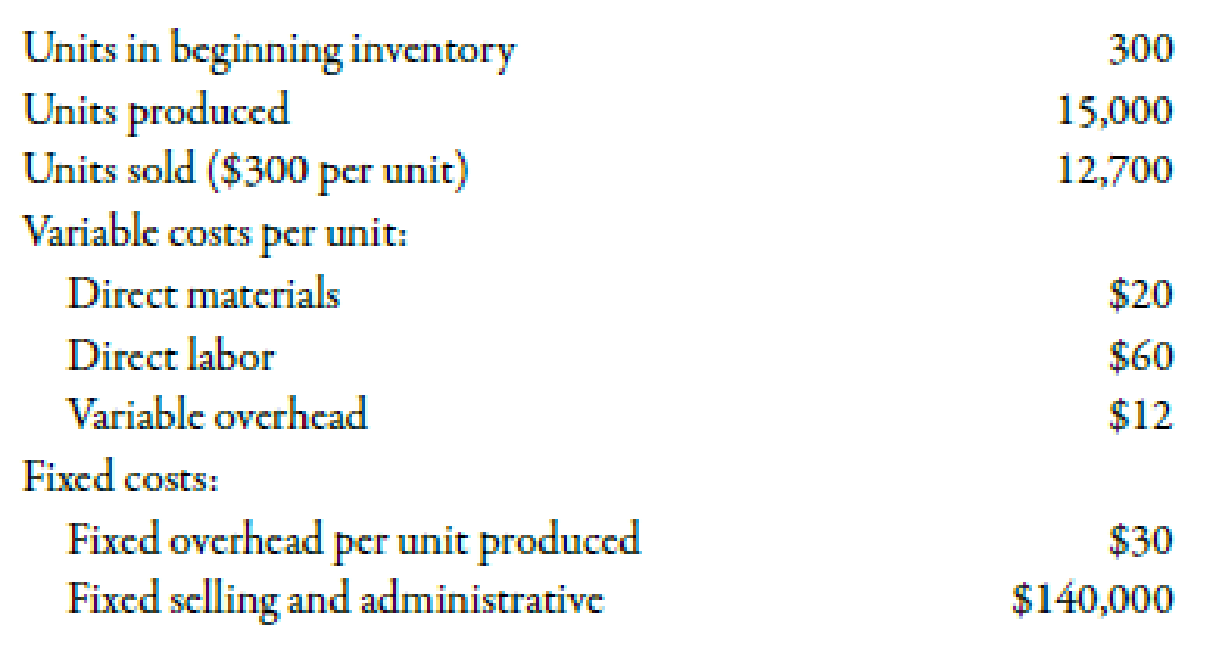Chapter 3, Problem 22BEA### Managerial Accounting: The Corners...

7th Edition
Maryanne M. Mowen + 2 others
ISBN: 9781337115773

#### Solutions

Chapter
Section### Managerial Accounting: The Corners...

7th Edition
Maryanne M. Mowen + 2 others
ISBN: 9781337115773
Textbook Problem
1 views

# Inventory Valuation under Variable CostingRefer to the data for Judson Company above.Required: 1. How many units are in ending inventory? 2. Using variable costing, calculate the per-unit product cost. 3. What is the value of ending inventory under variable costing? Use the following information for Brief Exercises 3-21 and 3-22:During the most recent year, Judson Company had the following data associated with the product it makes:1.

To determine

Calculate the ending inventory units.

Explanation

Variable Cost:

Variable cost is the cost which varies due to the changes in the level of output to produce products and services in a relevant range.

Use the following formula to calculate the value of ending inventory units:

Ending Inventory=Beggining Inventory+Units ProducedUnits Sold

Substitute 300 units for beginning inventory, 15,000 for units produced and 12,700 for units sold in the above formula

2.

To determine

Compute the per-unit product cost with the help of variable costing.

3.

To determine

Compute the value of ending inventory with the help of variable costing.

### Still sussing out bartleby?

Check out a sample textbook solution.

See a sample solution

#### The Solution to Your Study Problems

Bartleby provides explanations to thousands of textbook problems written by our experts, many with advanced degrees!

Get Started

#### Find more solutions based on key concepts